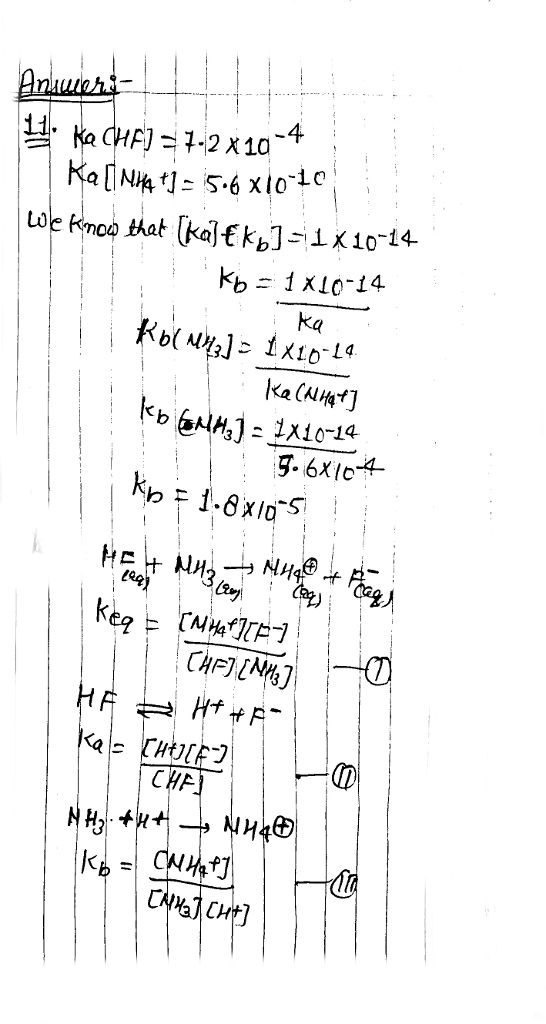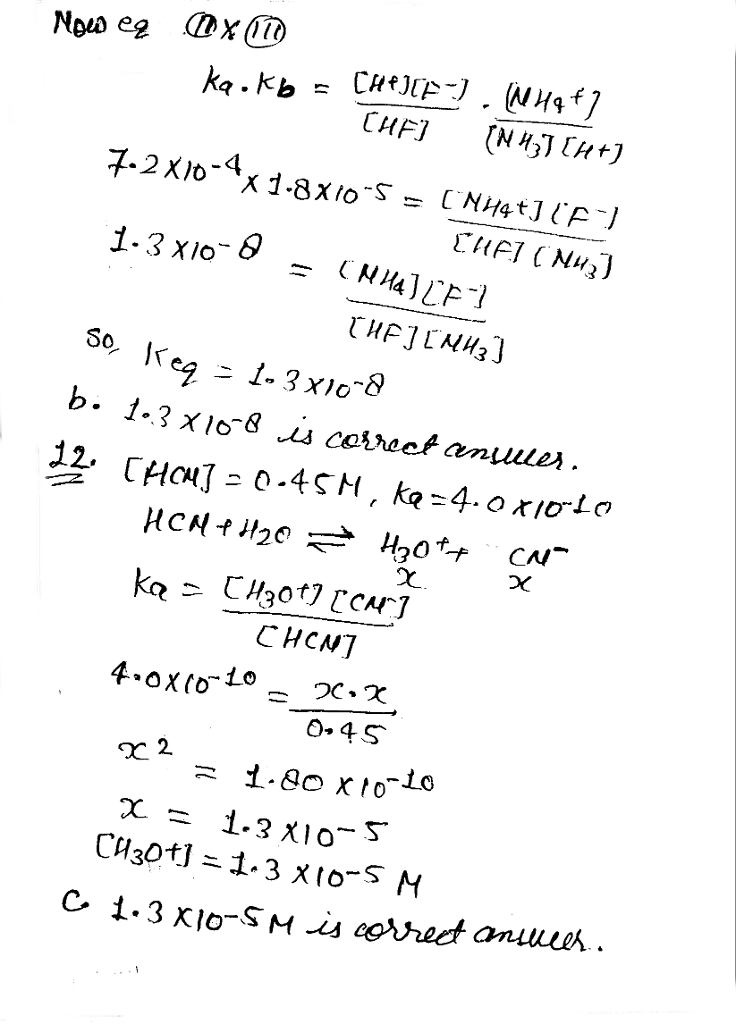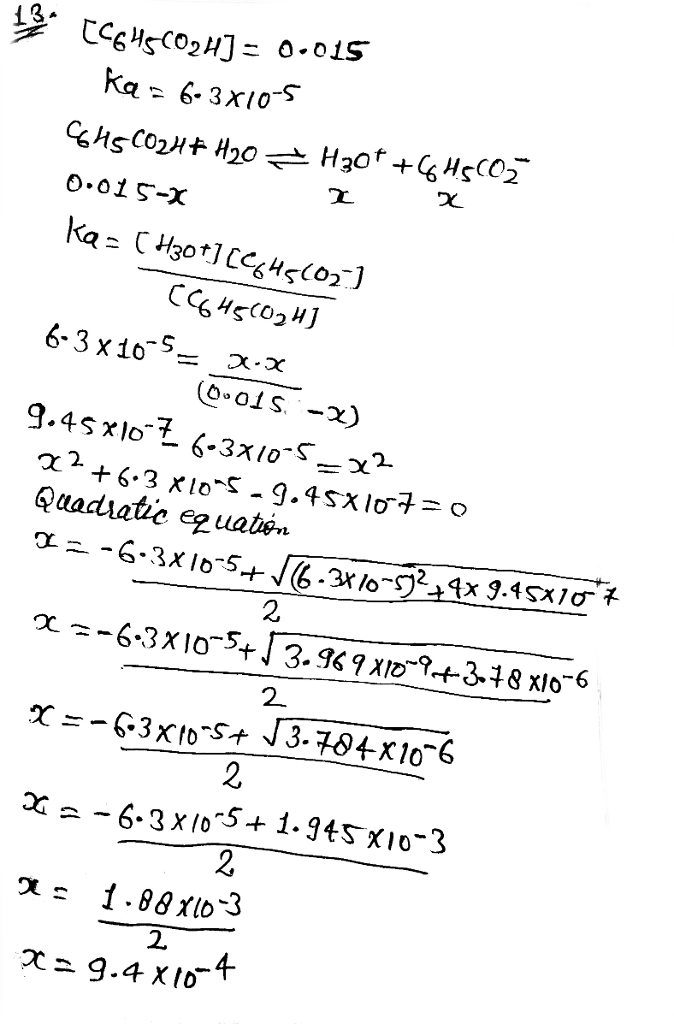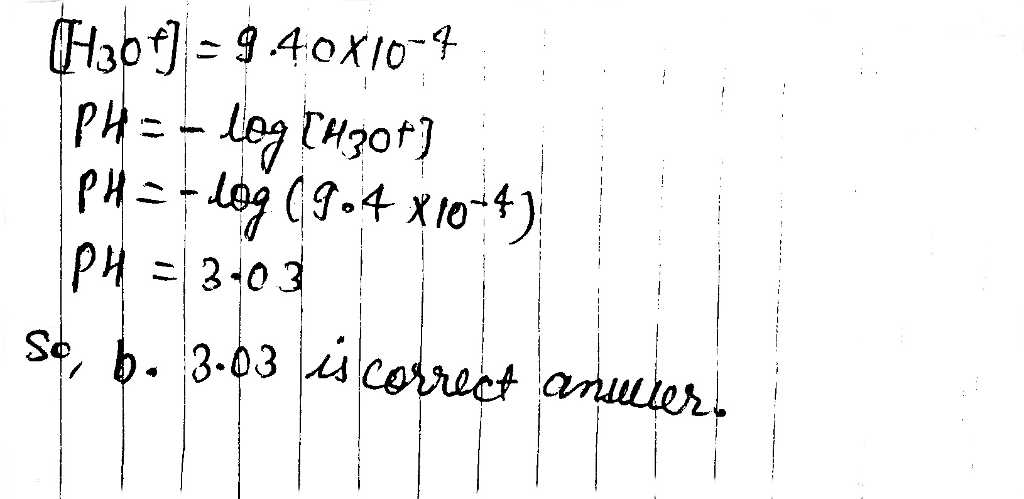# Question & Answer: Given the following acid dissociation constants, K_a (HF) = 7.2 times 10^-4 K_a…..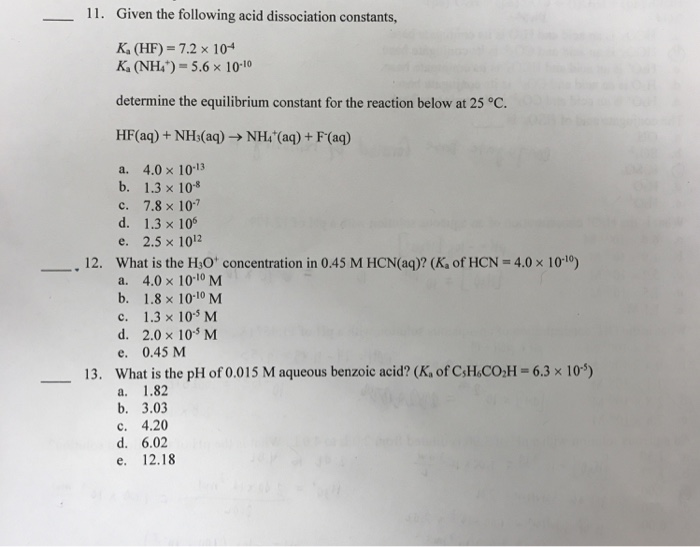Given the following acid dissociation constants, K_a (HF) = 7.2 times 10^-4 K_a (NH^+_4) = 5.6 times 10^-10 determine the equilibrium constant for the reaction below at 25 degree C. HF(aq) + NH_3(aq) rightarrow NH^+_4 (aq) + F^-(aq) a. 4.0 times 10^-13 b. 1.3 times 10^-8 c. 7.8 times 10^-7 d. 1.3 times 10^6 e. 2.5 times 10^12 What is the H_3O^+ concentration in 0.45 M HCN(aq)? (K_a of HCN = 4.0 times 10^-10) a. 4.0 times 10^-10 M b. 1.8 times 10^-10 M c. 1.3 times 10^-5 M d. 2.0 times 10^-5 M e. 0.45 M What is the pH of 0.015 M aqueous benzoic acid? (K_a of C_5H_6CO_2H = 6.3 times 10^-5) a. 1.82 b. 3.03 c. 4.20 d. 6.02 e. 12.18